# Restart – step by step: Read/Write SDRAM via Verilog

By | October 14, 2012

5 years ago, I was trying to design a CCD camera for astrophotography in  my spare time. But since I was major in Software Development, I didn't know much about hardware design other than 8051 MCU. After 1 year with many failures on wrong directions, I just clarified I'd better use CPLD or FPGA with Verilog/VHDL for sequential logic circuit instead of using a MCU everywhere. It was a hard progress. I had a goal too huge, and was eager for the result.

By making some tiny CPLD projects those years, I had a better understanding on sequential/combinational logic design using Verilog. I made a general experiment board for one of the projects, it mounted a Altera CPLD EPM570T100 and a Cypress 68013A USB client controller with 51 core. So I thought I can continue the camera designing now.

Last time, I was stopped by the RAM size limitation which should store a whole frame data of the Image sensor before sending via USB bulk protocol. So we begin by a little step: adding an external SDRAM to the system.

SDRAM has much complex timing diagram than SRAM. It has 6 operation stages for a simple single read: Active a "row", Wait For the "tRCD", send Read Command with a "column", PRECHARGE(means "DeActive"), Wait for the "CAS Latency", Read data.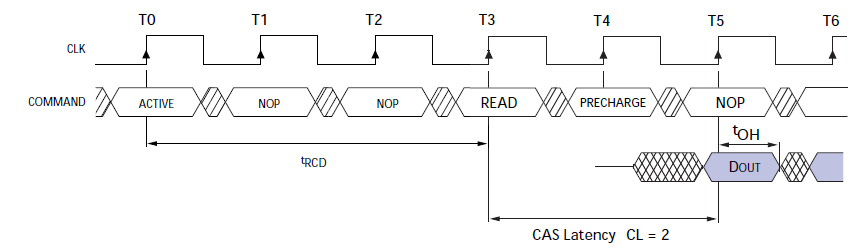tRCD is a const time related to the chip, my "H57V2562GTR-60C" has the tRCD equals to 18ns.

CAS Latency can be set during "Load Mode Register" stage. It can be 2 or 3 clock period.

More efficiently, there is "Brush Mode" for both reading and writing which can continuous read or write within a Active-Precharge period. But We are not going to discuss about it in this design.

I want to design a module to convert this complex interface into a more simple one. Operations which begin with a START signal on a rising-edge of the clock, need to wait for the FINISH signal only. So that a byte was read out or written into the SDRAM. The complex 6 stages would be hidden behind this module.

A FSM was created to handle this: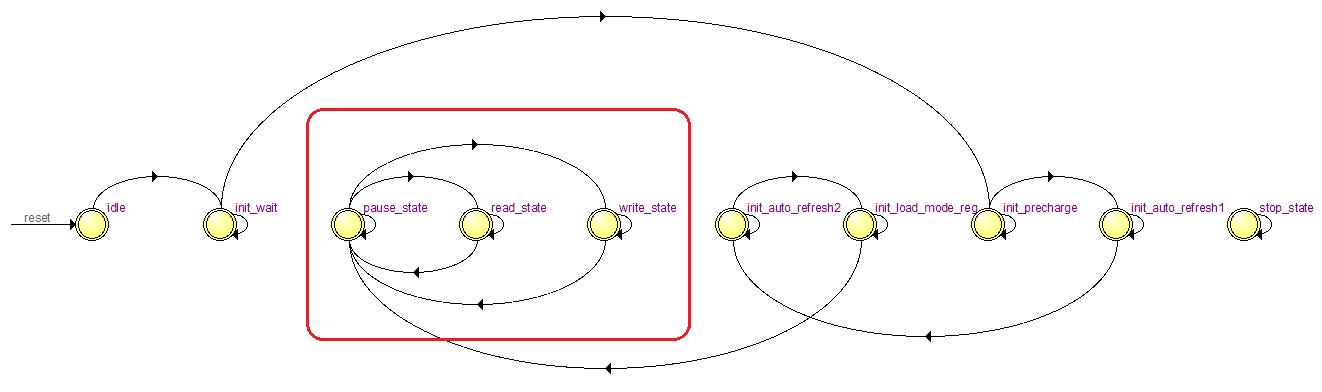The whole write operation simulated at gate level by ModelSim(Altera Edition):The read operation simulated at gate level: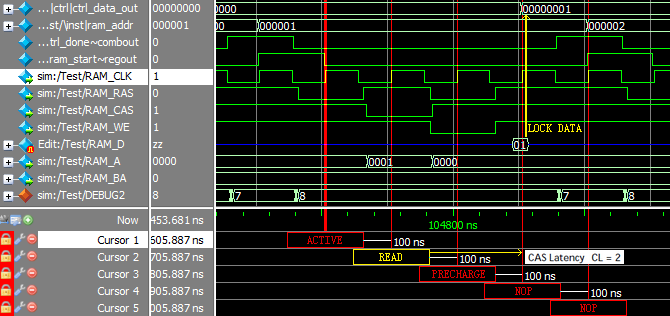Since I would operate the module at 10Mhz, the clock period is 100ns, the tRCD(18ns) is less than one clock time, so the NOP at T1 & T2 is not necessary.

My general experiment board with the SDRAM board mounted by half DIP sockets and half "jumper wires":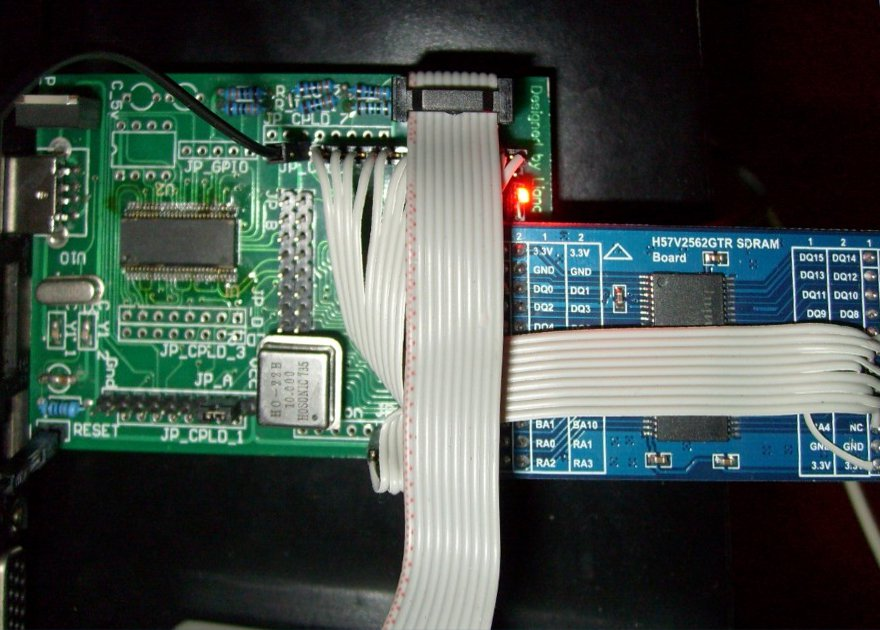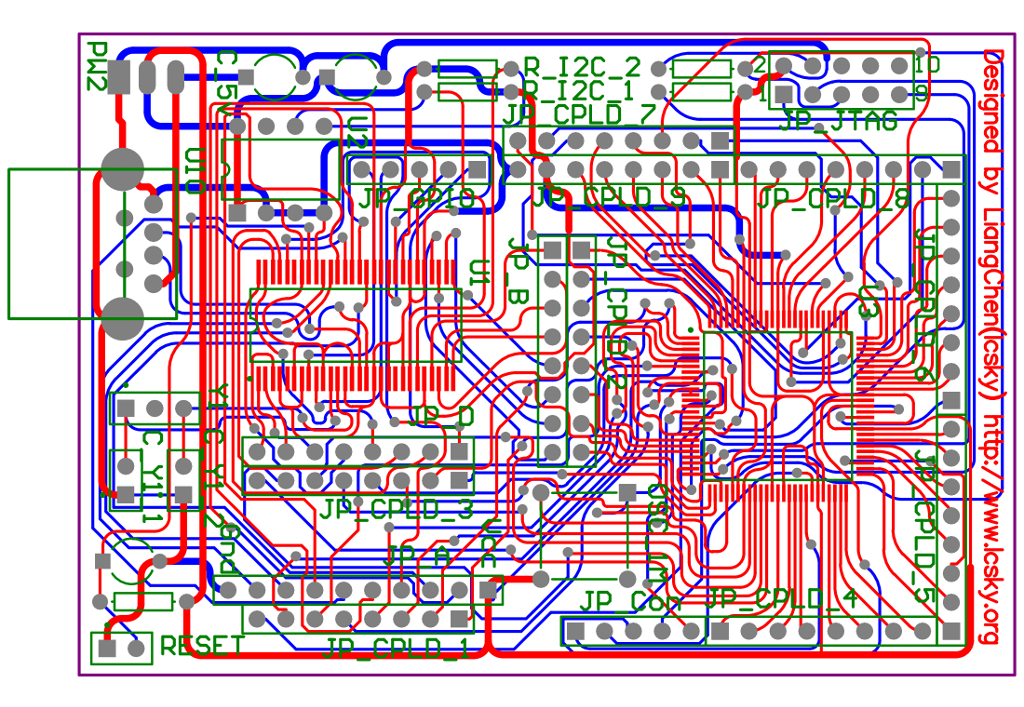Here is the code:

//Author: Lcsky.org
//Reference: http://www-inst.eecs.berkeley.edu/~cs150/fa02/handouts/10/Lab/
//Date: 2012/09/22

// Control module for SDRAM.
module sdram_ctrl (
RAM_D,				// to SDRAM
RAM_CLK,			// to SDRAM
RAM_DQM,			// to SDRAM
RAM_CS,				// to SDRAM
RAM_RAS,			// to SDRAM
RAM_CAS,			// to SDRAM
RAM_WE,				// to SDRAM
RAM_BA,				// to SDRAM
RAM_A,				// to SDRAM
CLK_IN, 			// Clock Line
CTRL_RESET_IN,	// Control Line
CTRL_START_IN,	// Control Line
CTRL_W_IN,			// Control Line
CTRL_DATA_IN,		// Control Line
CTRL_DATA_OUT,	// Control Line
CTRL_DONE_OUT,		// Control Line
DEBUG_OUT		// Control Line
);

inout[7:0] RAM_D;
output RAM_CLK;
output RAM_DQM;
output RAM_CS;
output RAM_RAS;
output RAM_CAS;
output RAM_WE;
output[1:0] RAM_BA;
output[12:0] RAM_A;
input CLK_IN;
input CTRL_RESET_IN;
input CTRL_START_IN;
input CTRL_W_IN;
input[7:0] CTRL_DATA_IN;
output[7:0] CTRL_DATA_OUT;
output CTRL_DONE_OUT;
output[3:0] DEBUG_OUT;

//Clock: 10M
parameter[31:0] CLK_SPEED = 10000000;

// counter for states
wire[15:0] count;
reg count_rst;

// current and nextstate
reg[3:0] CS;
reg[3:0] NS;

// different commands that can be issued to the SDRAM
reg NOP;
wire active;
reg write;
reg precharge;
reg autoPrecharge;
reg burstTerminate;
reg autoRefresh;
reg selfRefresh;
reg data_out_lock;

reg[7:0] ctrl_data_in;
reg[7:0] ctrl_data_out;
reg ctrl_done;

// mapping of commands to control lines Table1 p12 DATA sheet
assign RAM_RAS = ~(active | precharge |
autoRefresh | selfRefresh
assign RAM_CAS = ~(read | write |
autoRefresh | selfRefresh
assign RAM_WE = ~(write | burstTerminate |

assign RAM_CLK = ~CLK_IN;
assign RAM_CLKE = 1;
assign RAM_DQM = 0;
assign RAM_CS = 0;
assign DEBUG_OUT = CS;

assign active = active_write | active_read;

assign RAM_D = write ? ctrl_data_in : 8'bzzzzzzzz;

assign CTRL_DATA_OUT = ctrl_data_out;
assign CTRL_DONE_OUT = ctrl_done;

// into to 12bit address line
begin
else if (loadModeRegister) // mode data
addr_out = {3'b000, 1'b1 /* Single Burst */, 2'b00, 3'b010 /* CAS Latency = 2 */, 1'b0, 3'b000};
end
end

begin
else
end

counter #(16) state_counter(.OUT(count), .CLK(CLK_IN),
.ENABLE(1), .RESET(count_rst));

parameter[3:0]
idle = 0,
init_wait = 1,
init_precharge = 2,
init_auto_refresh1 = 3,
init_auto_refresh2 = 4,
pause_state = 7,
write_state = 9,
stop_state = 10;

always @ (posedge CLK_IN) begin
if (CTRL_RESET_IN) begin
CS <= idle;
end else begin
CS <= NS;
end
end

always @ (negedge CLK_IN) begin
if (data_out_lock && CS == read_state) begin
ctrl_data_out <= RAM_D;
end
end

always @(*) begin
NOP = 0;
active_write = 0;
write = 0;
precharge = 0;
autoPrecharge = 0;
burstTerminate = 0;
autoRefresh = 0;
selfRefresh = 0;
data_out_lock = 0;

count_rst = 0;

case (CS) /* synthesis full_case */

idle:begin
NS = init_wait;
count_rst = 1;
ctrl_done = 0;
end

init_wait:begin // nop for 100 micro sec
NOP = 1;
if (count == CLK_SPEED/10000) begin
NS = init_precharge;
count_rst = 1;
end else
NS = init_wait;
end

init_precharge:begin // 2 cycles (all banks): precharge, nop
if (count == 0) begin
precharge = 1;
NS = init_precharge;
end else begin
NOP = 1;
count_rst = 1;
NS = init_auto_refresh1;
end
end

init_auto_refresh1:begin // 8 cycles: auto-refresh, nopX7
if (count == 0) begin
autoRefresh = 1;
NS = init_auto_refresh1;
end else if (count == 7) begin
NOP = 1;
NS = init_auto_refresh2;
count_rst = 1;
end else begin
NOP = 1;
NS = init_auto_refresh1;
end
end

init_auto_refresh2:begin // 8 cycles: auto-refresh, nopX7
if (count == 0) begin
autoRefresh = 1;
NS = init_auto_refresh2;
end else if (count == 7) begin
NOP = 1;
count_rst = 1;
end else begin
NOP = 1;
NS = init_auto_refresh2;
end
end

if (count == 0) begin
end else begin
NOP = 1;
count_rst = 1;
NS = pause_state;
end
end

pause_state: begin // waiting for CTRL_START_IN
NOP = 1;
if (CTRL_START_IN) begin
NS = CTRL_W_IN ? write_state : read_state;
count_rst = 1;

end else begin
NS = pause_state;
ctrl_done = 1;
end
end

write_state: begin // 3 cycles: active, write, precharge
NS = write_state;
if (count == 0) begin
ctrl_data_in = CTRL_DATA_IN;
ctrl_done = 0;

active_write = 1;
end else if (count == 1) begin
write = 1;
end else begin
count_rst = 1;
precharge = 1;
NS = pause_state;
end
end

if (count == 0) begin
ctrl_done = 0;

end else if (count == 1) begin
end else if (count == 2) begin
precharge = 1;
end else begin
// trigger another "always block" to read data in half period later.
data_out_lock = 1;

NOP = 1;
count_rst = 1;
NS = pause_state;
end
end

stop_state: begin // stop
NOP = 1;
count_rst = 1;
NS = stop_state;
end
endcase
end
endmodule